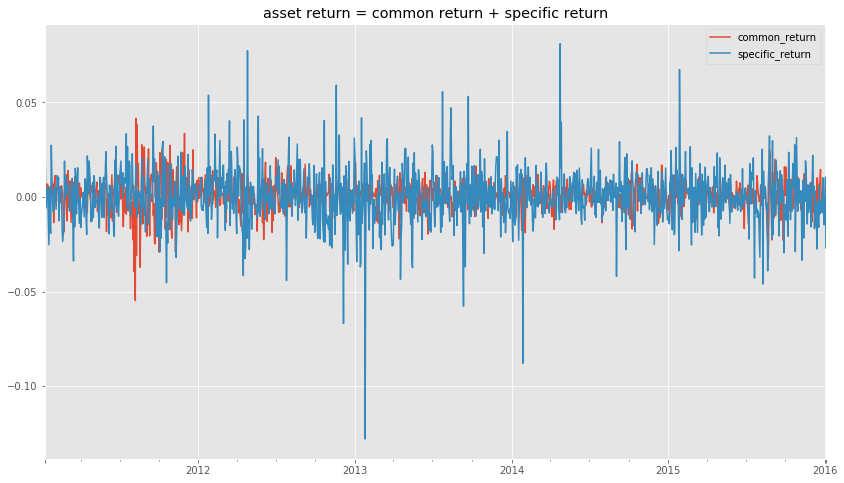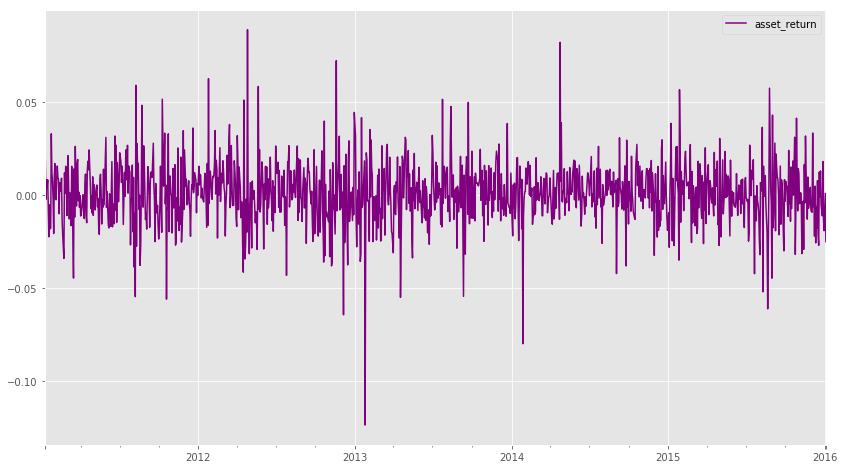# AI For Trading: Factor Model of Asset Return (52)

## Factor Model of Return

An asset's return can be modeled as the contribution from a set of factors, plus the specific return (the part that isn't explained by the factors).

## Factor Model of Asset Return Exercise

Factor Model of Asset Return

import sys
!{sys.executable} -m pip install -r requirements.txt
Requirement already satisfied: numpy==1.14.5 in /opt/conda/lib/python3.6/site-packages (from -r requirements.txt (line 1))
Requirement already satisfied: pandas==0.18.1 in /opt/conda/lib/python3.6/site-packages (from -r requirements.txt (line 2))
Requirement already satisfied: plotly==2.2.3 in /opt/conda/lib/python3.6/site-packages (from -r requirements.txt (line 3))
Requirement already satisfied: scikit-learn==0.19.1 in /opt/conda/lib/python3.6/site-packages (from -r requirements.txt (line 4))
Requirement already satisfied: six==1.11.0 in /opt/conda/lib/python3.6/site-packages (from -r requirements.txt (line 5))
Requirement already satisfied: zipline===1.2.0 in /opt/conda/lib/python3.6/site-packages (from -r requirements.txt (line 6))
Requirement already satisfied: python-dateutil>=2 in /opt/conda/lib/python3.6/site-packages (from pandas==0.18.1->-r requirements.txt (line 2))
Requirement already satisfied: pytz>=2011k in /opt/conda/lib/python3.6/site-packages (from pandas==0.18.1->-r requirements.txt (line 2))
Requirement already satisfied: decorator>=4.0.6 in /opt/conda/lib/python3.6/site-packages (from plotly==2.2.3->-r requirements.txt (line 3))
Requirement already satisfied: requests in /opt/conda/lib/python3.6/site-packages (from plotly==2.2.3->-r requirements.txt (line 3))
Requirement already satisfied: nbformat>=4.2 in /opt/conda/lib/python3.6/site-packages (from plotly==2.2.3->-r requirements.txt (line 3))
Requirement already satisfied: intervaltree>=2.1.0 in /opt/conda/lib/python3.6/site-packages (from zipline===1.2.0->-r requirements.txt (line 6))
Requirement already satisfied: MarkupSafe>=0.23 in /opt/conda/lib/python3.6/site-packages (from zipline===1.2.0->-r requirements.txt (line 6))
Requirement already satisfied: contextlib2>=0.4.0 in /opt/conda/lib/python3.6/site-packages (from zipline===1.2.0->-r requirements.txt (line 6))
Requirement already satisfied: empyrical>=0.4.2 in /opt/conda/lib/python3.6/site-packages (from zipline===1.2.0->-r requirements.txt (line 6))
Requirement already satisfied: numexpr>=2.6.1 in /opt/conda/lib/python3.6/site-packages (from zipline===1.2.0->-r requirements.txt (line 6))
[33mYou are using pip version 9.0.1, however version 18.1 is available.
You should consider upgrading via the 'pip install --upgrade pip' command.[0m
import numpy as np
import pandas as pd
import time
import os
import quiz_helper
import matplotlib.pyplot as plt
%matplotlib inline
plt.style.use('ggplot')
plt.rcParams['figure.figsize'] = (14, 8)

### data bundle

import os
import quiz_helper
from zipline.data import bundles
os.environ['ZIPLINE_ROOT'] = os.path.join(os.getcwd(), '..', '..','data','module_4_quizzes_eod')
ingest_func = bundles.csvdir.csvdir_equities(['daily'], quiz_helper.EOD_BUNDLE_NAME)
bundles.register(quiz_helper.EOD_BUNDLE_NAME, ingest_func)
print('Data Registered')
Data Registered

### Build pipeline engine

from zipline.pipeline import Pipeline
from zipline.pipeline.factors import AverageDollarVolume
from zipline.utils.calendars import get_calendar

universe = AverageDollarVolume(window_length=120).top(500)
engine = quiz_helper.build_pipeline_engine(bundle_data, trading_calendar)

### View Data露

With the pipeline engine built, let's get the stocks at the end of the period in the universe we're using. We'll use these tickers to generate the returns data for the our risk model.

universe_end_date = pd.Timestamp('2016-01-05', tz='UTC')

universe_tickers = engine\
.run_pipeline(
Pipeline(screen=universe),
universe_end_date,
universe_end_date)\
.index.get_level_values(1)\
.values.tolist()

universe_tickers
[Equity(0 [A]),
Equity(1 [AAL]),
Equity(2 [AAP]),
Equity(3 [AAPL]),
Equity(4 [ABBV]),
Equity(5 [ABC]),
Equity(6 [ABT]),
Equity(7 [ACN]),
Equity(14 [AEE]),
Equity(15 [AEP]),
Equity(16 [AES]),
Equity(17 [AET]),
Equity(18 [AFL]),
Equity(19 [AGN]),
Equity(20 [AIG]),
Equity(21 [AIV]),
Equity(22 [AIZ]),
Equity(23 [AJG]),
Equity(24 [AKAM]),
Equity(25 [ALB]),
Equity(26 [ALGN]),
Equity(27 [ALK]),
Equity(28 [ALL]),
Equity(29 [ALLE]),
Equity(30 [ALXN]),
Equity(31 [AMAT]),
Equity(32 [AMD]),
Equity(33 [AME]),
Equity(34 [AMG]),
Equity(35 [AMGN]),
Equity(36 [AMP]),
Equity(37 [AMT]),
Equity(38 [AMZN]),
Equity(39 [ANDV]),
Equity(40 [ANSS]),
Equity(41 [ANTM]),
Equity(42 [AON]),
Equity(43 [AOS]),
Equity(44 [APA]),
Equity(45 [APC]),
Equity(46 [APD]),
Equity(47 [APH]),
Equity(48 [ARE]),
Equity(49 [ARNC]),
Equity(50 [ATVI]),
Equity(51 [AVB]),
Equity(52 [AVGO]),
Equity(53 [AVY]),
Equity(54 [AWK]),
Equity(55 [AXP]),
Equity(56 [AYI]),
Equity(57 [AZO]),
Equity(58 [BA]),
Equity(59 [BAC]),
Equity(60 [BAX]),
Equity(61 [BBT]),
Equity(62 [BBY]),
Equity(63 [BCR]),
Equity(64 [BDX]),
Equity(65 [BEN]),
Equity(66 [BIIB]),
Equity(67 [BK]),
Equity(68 [BLK]),
Equity(69 [BLL]),
Equity(70 [BMY]),
Equity(71 [BSX]),
Equity(72 [BWA]),
Equity(73 [BXP]),
Equity(74 [C]),
Equity(75 [CA]),
Equity(76 [CAG]),
Equity(77 [CAH]),
Equity(78 [CAT]),
Equity(79 [CB]),
Equity(80 [CBG]),
Equity(81 [CBOE]),
Equity(82 [CBS]),
Equity(83 [CCI]),
Equity(84 [CCL]),
Equity(85 [CELG]),
Equity(86 [CERN]),
Equity(87 [CF]),
Equity(88 [CFG]),
Equity(89 [CHD]),
Equity(90 [CHK]),
Equity(91 [CHRW]),
Equity(92 [CHTR]),
Equity(93 [CI]),
Equity(94 [CINF]),
Equity(95 [CL]),
Equity(96 [CLX]),
Equity(97 [CMA]),
Equity(98 [CMCSA]),
Equity(99 [CME]),
Equity(100 [CMG]),
Equity(101 [CMI]),
Equity(102 [CMS]),
Equity(103 [CNC]),
Equity(104 [CNP]),
Equity(105 [COF]),
Equity(106 [COG]),
Equity(107 [COL]),
Equity(108 [COO]),
Equity(109 [COP]),
Equity(110 [COST]),
Equity(111 [COTY]),
Equity(112 [CPB]),
Equity(113 [CRM]),
Equity(114 [CSCO]),
Equity(115 [CSRA]),
Equity(116 [CSX]),
Equity(117 [CTAS]),
Equity(118 [CTL]),
Equity(119 [CTSH]),
Equity(120 [CTXS]),
Equity(121 [CVS]),
Equity(122 [CVX]),
Equity(123 [CXO]),
Equity(124 [D]),
Equity(125 [DAL]),
Equity(126 [DE]),
Equity(127 [DFS]),
Equity(128 [DG]),
Equity(129 [DGX]),
Equity(130 [DHI]),
Equity(131 [DHR]),
Equity(132 [DIS]),
Equity(133 [DISCA]),
Equity(134 [DISCK]),
Equity(135 [DISH]),
Equity(136 [DLR]),
Equity(137 [DLTR]),
Equity(138 [DOV]),
Equity(139 [DPS]),
Equity(140 [DRE]),
Equity(141 [DRI]),
Equity(142 [DTE]),
Equity(143 [DUK]),
Equity(144 [DVA]),
Equity(145 [DVN]),
Equity(146 [EA]),
Equity(147 [EBAY]),
Equity(148 [ECL]),
Equity(149 [ED]),
Equity(150 [EFX]),
Equity(151 [EIX]),
Equity(152 [EL]),
Equity(153 [EMN]),
Equity(154 [EMR]),
Equity(155 [EOG]),
Equity(156 [EQIX]),
Equity(157 [EQR]),
Equity(158 [EQT]),
Equity(159 [ES]),
Equity(160 [ESRX]),
Equity(161 [ESS]),
Equity(162 [ETFC]),
Equity(163 [ETN]),
Equity(164 [ETR]),
Equity(165 [EVHC]),
Equity(166 [EW]),
Equity(167 [EXC]),
Equity(168 [EXPD]),
Equity(169 [EXPE]),
Equity(170 [EXR]),
Equity(171 [F]),
Equity(172 [FAST]),
Equity(173 [FB]),
Equity(174 [FBHS]),
Equity(175 [FCX]),
Equity(176 [FDX]),
Equity(177 [FE]),
Equity(178 [FFIV]),
Equity(179 [FIS]),
Equity(180 [FISV]),
Equity(181 [FITB]),
Equity(182 [FL]),
Equity(183 [FLIR]),
Equity(184 [FLR]),
Equity(185 [FLS]),
Equity(186 [FMC]),
Equity(187 [FOX]),
Equity(188 [FOXA]),
Equity(189 [FRT]),
Equity(190 [FTI]),
Equity(191 [GD]),
Equity(192 [GE]),
Equity(193 [GGP]),
Equity(194 [GILD]),
Equity(195 [GIS]),
Equity(196 [GLW]),
Equity(197 [GM]),
Equity(198 [GOOG]),
Equity(199 [GOOGL]),
Equity(200 [GPC]),
...
Equity(488 [ZBH]),
Equity(489 [ZION]),
Equity(490 [ZTS])]
len(universe_tickers)
490
from zipline.data.data_portal import DataPortal

data_portal = DataPortal(
bundle_data.asset_finder,
adjustment_reader=bundle_data.adjustment_reader)

## Get pricing data helper function

from quiz_helper import get_pricing

## get pricing data into a dataframe

returns_df = \
get_pricing(
data_portal,
universe_tickers,
universe_end_date - pd.DateOffset(years=5),
universe_end_date)\
.pct_change()[1:].fillna(0) #convert prices into returns

returns_df
Equity(0 [A]) Equity(1 [AAL]) Equity(2 [AAP]) Equity(3 [AAPL]) Equity(4 [ABBV]) Equity(5 [ABC]) Equity(6 [ABT]) Equity(7 [ACN]) Equity(8 [ADBE]) Equity(9 [ADI]) ... Equity(481 [XL]) Equity(482 [XLNX]) Equity(483 [XOM]) Equity(484 [XRAY]) Equity(485 [XRX]) Equity(486 [XYL]) Equity(487 [YUM]) Equity(488 [ZBH]) Equity(489 [ZION]) Equity(490 [ZTS])
2011-01-07 00:00:00+00:00 0.008437 0.014230 0.026702 0.007146 0.000000 0.001994 0.004165 0.001648 -0.007127 -0.005818 ... -0.001838 -0.005619 0.005461 -0.004044 -0.013953 0.000000 0.012457 -0.000181 -0.010458 0.000000
2011-01-10 00:00:00+00:00 -0.004174 0.006195 0.007435 0.018852 0.000000 -0.005714 -0.008896 -0.008854 0.028714 0.002926 ... 0.000947 0.007814 -0.006081 0.010466 0.009733 0.000000 0.001440 0.007784 -0.017945 0.000000
2011-01-11 00:00:00+00:00 -0.001886 -0.043644 -0.005927 -0.002367 0.000000 0.009783 -0.002067 0.013717 0.000607 0.008753 ... 0.001314 0.010179 0.007442 0.007351 0.006116 0.000000 -0.006470 0.035676 0.007467 0.000000
2016-01-05 00:00:00+00:00 0.004058 -0.009541 -0.006830 -0.025054 -0.004169 0.014629 -0.000247 0.005207 0.004023 -0.007347 ... 0.002098 0.014863 0.008511 0.020390 -0.001957 -0.000286 -0.002495 0.020820 -0.010853 0.015647

1256 rows 脳 490 columns

## Let's look at one stock

Let's look at this for just one stock. We'll pick AAPL in this example.

aapl_col = returns_df.columns
asset_return = returns_df[aapl_col]

asset_return = asset_return.rename('asset_return')

## Factor returns

Let's make up a "factor" by taking an average of all stocks in our list. You can think of this as an equal weighted index of the 490 stocks, kind of like a measure of the "market". We'll also make another factor by calculating the median of all the stocks. These are mainly intended to help us generate some data to work with. We'll go into how some common risk factors are generated later in the lessons.

Also note that we're setting axis=1 so that we calculate a value for each time period (row) instead of one value for each column (assets).

factor_return_1 = returns_df.mean(axis=1)
factor_return_2 = returns_df.median(axis=1)

## Factor exposures

Factor exposures refer to how "exposed" a stock is to each factor. We'll get into this more later. For now, just think of this as one number for each stock, for each of the factors.

from sklearn.linear_model import LinearRegression
"""
You can run these in separate cells to see each step in detail
But for now, just assume that we're calculating a number for each
stock, for each factor, which represents how "exposed" each stock is
to each factor.
We'll discuss how factor exposure is calculated later in the lessons.
"""
lr = LinearRegression()
X = np.array([factor_return_1.values,factor_return_2.values]).T
y = np.array(asset_return.values)
lr.fit(X,y)
factor_exposure_1 = lr.coef_
factor_exposure_2 = lr.coef_

## Quiz 1 Contribution of Factors

The sum of the products of factor exposure times factor return is the contribution of the factors. It's also called the "common return." calculate the common return of AAPL, given the two factor exposures and the two factor returns.

# Calculate the contribution of the two factors to the return of this example asset
common_return = (factor_exposure_1 * factor_return_1) + (factor_exposure_2 * factor_return_2)
common_return = common_return.rename('common_return')

## Quiz 2 Specific Return

The specific return is the part of the stock return that isn't explained by the factors. So it's the actual return minus the common return.
Calculate the specific return of the stock.

# TODO: calculate the specific return of this asset
specific_return = asset_return - common_return

specific_return = specific_return.rename('specific_return')

## Visualize the common return and specific return

return_components = pd.concat([common_return,specific_return],axis=1)
return_components.head(2)
common_return specific_return
2011-01-07 00:00:00+00:00 0.000654 0.006492
2011-01-10 00:00:00+00:00 0.000506 0.018346
return_components.plot(title="asset return = common return + specific return");
pd.DataFrame(asset_return).plot(color='purple');